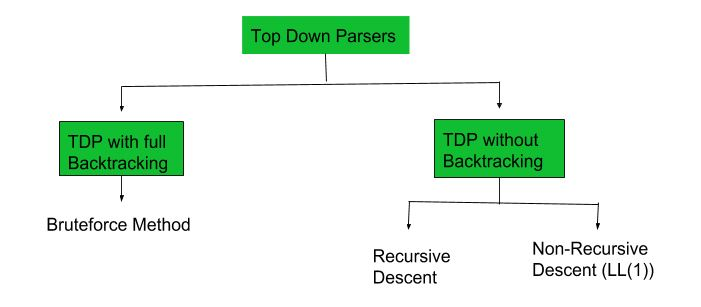# Compiler Design | Construction of LL(1) Parsing Table

Prerequisite – Classification of top down parsers, FIRST Set, FOLLOW Set
A top-down parser builds the parse tree from the top down, starting with the start non-terminal. There are two types of Top Down Parsers:

1. Top Down Parser with Backtracking
2. Top Down Parsers without Backtracking

Top Down Parsers without Backtracking can further be divided into two parts:In this article we are going to discuss about Non-Recursive Descent which is also known as LL(1) Parser.

LL(1) Parsing:
Here the 1st L represents that the scanning of the Input will be done from Left to Right manner and second L shows that in this Parsing technique we are going to use Left most Derivation Tree. and finally the 1 represents the number of look ahead, means how many symbols are you going to see when you want to make a decision.

Construction of LL(1) Parsing Table:
To construct the Parsing table, we have two functions:

1: First(): If there is a variable, and from that variable if we try to drive all the strings then the beginning Terminal Symbol is called the first.

2: Follow(): What is the Terminal Symbol which follow a variable in the process of derivation.

Now, after computing the First and Follow set for each Non-Terminal symbol we have to construct the Parsing table. In the table Rows will contain the Non-Terminals and the column will contain the Terminal Symbols.
All the Null Productions of the Grammars will go under the Follow elements and the remaining productions will lie under the elements of First set.

Now, let’s understand with an example.

Example-1:
Consider the Grammar:

```E --> TE'
E' --> +TE' | e
T --> FT'
T' --> *FT' | e
F --> id | (E)

**e denotes epsilon```

Find their first and follow sets:

First Follow
E –> TE’ { id, ( } { \$, ) }
E’ –> +TE’/e { +, e } { \$, ) }
T –> FT’ { id, ( } { +, \$, ) }
T’ –> *FT’/e { *, e } { +, \$, ) }
F –> id/(E) { id, ( } { *, +, \$, ) }

Now, the LL(1) Parsing Table is:

id + * ( ) \$
E E –> TE’ E –> TE’
E’ E’ –> +TE’ E’ –> e E’ –> e
T T –> FT’ T –> FT’
T’ T’ –> e T’ –> *FT T’ –> e T’ –> e
F F –> id F –> (E)

As you can see that all the null productions are put under the follow set of that symbol and all the remaining productions are lie under the first of that symbol.

Note: Every grammar is not feasible for LL(1) Parsing table. It may be possible that one cell may contain more than one production.

Let’s see with an example.

Example-2:
Consider the Grammar

```S --> A | a
A --> a ```

Find their first and follow sets:

First Follow
S –> A/a { a } { \$ }
A –>a { a } { \$ }

Parsing Table:

a \$
S S –> A, S –> a
A A –> a

Here, we can see that there are two productions into the same cell. Hence, this grammar is not feasible for LL(1) Parser.My Personal Notes arrow_drop_upCheck out this Author's contributed articles.

If you like GeeksforGeeks and would like to contribute, you can also write an article using contribute.geeksforgeeks.org or mail your article to contribute@geeksforgeeks.org. See your article appearing on the GeeksforGeeks main page and help other Geeks.

Please Improve this article if you find anything incorrect by clicking on the "Improve Article" button below.

Article Tags :

6

Please write to us at contribute@geeksforgeeks.org to report any issue with the above content.